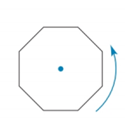Chapter 2.6, Problem 32E### Elementary Geometry for College St...

6th Edition
Daniel C. Alexander + 1 other
ISBN: 9781285195698

#### Solutions

Chapter
Section### Elementary Geometry for College St...

6th Edition
Daniel C. Alexander + 1 other
ISBN: 9781285195698
Textbook Problem
17 views

# A regular octagon is rotated about acentrally located point (as shown). Howmany rotations are needed to repeat thegiven octagon, vertex for vertex, if theangle of rotation isa) 10 ° b) 45 ° c) 90 ° d) 120 °

To determine

a)

To find:

The rotations needed to repeat the octagon vertex-for vertex.

Explanation

Given:

The angle of rotation is 10°.

The given figure is,

Figure (1)

Property:

The octagon repeats vertex-for vertex if it is rotated for angle of 45° or its multiple angle such as 90°, and 180°, 360°

To determine

b)

To find:

The rotations needed to repeat the octagon vertex-for vertex.

To determine

c)

To find:

The rotations needed to repeat the octagon vertex-for vertex.

To determine

d)

To find:

The rotations needed to repeat the octagon vertex-for vertex.

### Still sussing out bartleby?

Check out a sample textbook solution.

See a sample solution

#### The Solution to Your Study Problems

Bartleby provides explanations to thousands of textbook problems written by our experts, many with advanced degrees!

Get Started

#### If f is continuous and 04f(x)dx=10, find 02f(2x)dx.

Single Variable Calculus: Early Transcendentals, Volume I

#### In Exercises 13-20, sketch a set of coordinate axes and plot each point. 16. (3, 4)

Applied Calculus for the Managerial, Life, and Social Sciences: A Brief Approach

#### For f(x)=exx,f(x)= a) ex b) xex1x2 c) exxexx2 d) xexxx2

Study Guide for Stewart's Single Variable Calculus: Early Transcendentals, 8th